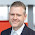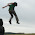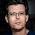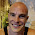# The 20% Statistician

A blog on statistics, methods, philosophy of science, and open science. Understanding 20% of statistics will improve 80% of your inferences.

## Monday, June 8, 2015

### Why you should use omega-squared instead of eta-squared.

[The points made in this blog posts are now published: Albers, C. & Lakens, D. (2017). Biased sample size estimates in a-priori power analysis due to the choice of the effect size index and follow-up bias. Journal of Experimental Social Psychology. Read the pre-print here.]

Eta-squared (η²) and partial eta-squared (ηp²) are biased effect size estimators. I knew this, but I never understood how bad it was. Here’s how bad it is: If η² was a flight from New York to Amsterdam, you would end up in Berlin. Because of the bias, using η² or ηp² in power analyses can lead to underpowered studies, because the sample size estimate will be too small. Below, I’ll share a relatively unknown but remarkably easy to use formula to calculate partial omega squared ωp² which you can use in power analyses instead of ηp². You should probably always us ωp² in power analyses. And it makes sense to round ωp² to three digits instead of two, especially for small to medium effect sizes.

Effect sizes have variance (they vary every time you would perform the same experiment) but they can also have systematic bias. For Cohen’s d a less biased effect size estimate is known as Hedges’ g. For η² less biased estimators are epsilon squared (ε²) and omega-squared (ω²). Texts on statistics often mention ω² is a less biased version of η², but I’ve never heard people argue strongly against using η² at all (EDIT: This was probably because I hadn't read Caroll & Nordholm, 1975; Skidmore & Thompson, 2012;  Wickens & Keppel, 2004. For example, in Skidmore & Thompson, 2012: "Overall, our results corroborate the limited previous research (Carroll & Nordholm, 1975; Keselman, 1975) and suggest that η2 should not be used as an ANOVA effect size estimator"). Because no one ever clearly demonstrated to me how much it matters, and software such as SPSS conveniently provides ηp² but not εp² or ωp², I personally ignored ω². I thought that if it really mattered, we would all be using ω². Ha, ha.

While reading up on this topic, I came across work by Okada (2013). He re-examines the widespread belief that ω² is the preferred and least biased alternative to η². Keselman (1975) had shown that in terms of bias ω² < ε² < η². It turns out that might have been due to a lousy random number generator and too small sample size (number of simulations). Okada (2013) shows that in terms of bias ε² < ω² < η². It demonstrates that even in statistics replication is really important.

The bias in η² decreases as the sample size per condition increases, and it increases as the effect size becomes smaller (but not that much). Because the size of the bias remains rather stable as the true effect size decreases, the ratio between the bias and the true effect size becomes larger when the effect size decreases. Where an overestimation of 0.03 isn’t huge when η² = 0.26, it is substantial when the true η² = 0.06. Let’s see how much it matters, for all practical purposes.

How biased is eta-squared?

Okada (2013) includes a table with the bias for η², ε², and ω², for sample sizes of 10 to 100 per condition, for three effect sizes. He uses small, medium, and large effect sizes following Keselman (1975), but I have run additional simulations for the now more commonly used small (η² = 0.0099), medium (η² = 0.0588), and large (η² = 0.1379) effects, based on Cohen (1988). Cohen actually meant ηp² with these benchmarks (as Richardson, 2011 recently reminded me), but in a One-Way ANOVA η² = ηp². I’ve used Okada’s R script to calculate the bias for four effect sizes (based on 1000000 simulations): no effect (η² = 0), small (η² = 0.0099), medium (η² = 0.0588), and large (η² = 0.1379). Note therefore that my labels for small, medium, and large differ from those in Okada (2013). Also, formula 5 for ω² in Okada (2013) is incorrect, the denominator should be SSt/MSw instead of SSt/SSw. It is a typo - the correct formula was used in the R script. The script simulated an ANOVA with 4 groups (click to enlarge).

The table shows the bias. With four groups of n = 20, a One-Way ANOVA with a medium effect (true η² = 0.0588) will overestimate the true effect size on average by 0.03512, for an average observed η² of = 0.0588 + 0.03512 = 0.09392. We can see that for small effects (η² = 0.0099) the bias is actually larger than the true effect size (up to ANOVA’s with 70 participants in each condition).

When there is no true effect, η² from small studies can easily give the wrong impression that there is a real small to medium effect, just due to the bias. Your p-value would not be statistically significant, but this overestimation could be problematic if you ignore the p-value and just focus on estimation.

I ran some additional simulations with two groups (see the Table below). The difference between the 4 group and 2 group simulations shows the bias increases as the number of groups increases. It is smallest with only two groups, but even here, the eta-squared from studies with small samples are biased and this bias will influence power calculations. It is also important to correct for this bias in meta-analysis. Instead of converting η² directly to r, it is better to convert ω² to r. It would be interesting to examine if this bias influences published meta-analyses.

It is also clear that ε² and ω² are massively more accurate. As Okada (2013) observed, and unlike most statistics textbook will tell you, ε² is less biased than ω². However, they both do a good job for most practical purposes. Based on the belief ω² was less biased than ε², statisticians typically ignore ε². For example, (Olejnik & Algina, 2003) discuss generalized ωG², but not εG². More future work.

Impact on A-Priori Power Analysis

Let’s say you perform a power analysis using the observed η² of 0.0935 when the true η² = 0.0588. With 80% power, an alpha of 0.05, and 4 groups, the recommended sample size is 112 participants in total. An a-priori power analysis with the correct (true) η² = 0.0588 would have yielded a required sample size of 180. With 112 participants, you would have 57% power, instead of 80% power.

So there’s that.

Even when the bias in η² is only 0.01, we are still talking about a sample size calculation of 152 instead of 180 for a medium effect size. If you consider the fact we are only reporting this effect size because SPSS gives it, we might just as well report something more useful. I think as scientists we should not run eta-airways with flights from New-York to Amsterdam that end up in Berlin. We should stop using eta-squared. (Obviously, it would help if statistic software would report unbiased effect sizes. The only statistical software I know that has an easy way to request omega-squared is Stata – even R fails us here - but see the easy to use formula below).

This also means it makes sense to round ηp² or ωp² to three digits instead of two. When you observe a medium effect, and write down that ωp² = 0.06, it matters for a power analysis (2 groups, 80% power) whether the true value was 0.064 (total sample size: 118) or 0.056 (total sample size: 136). The difference becomes more pronounced for smaller effect sizes. Obviously, power analyses at their best give a tentative answer to the number of participants you need. Sequential analyses are a superior way to design well-powered studies.

Calculating Partial Omega-Squared

Here’s the game-changer for me, personally. In power analysis, you need the partial effect size. I thought you could only calculate ωp² if you had access to the ANOVA table, because most formulas look like this:

and the mean squares are not available to researchers. But, Maxwell and Delaney (2004, formula 7.46)  apply some basic algebra and give a much more useful formula to calculate ωp² EDIT: Even more useful are the formula's by Carroll and Nordholm (1975) (only to be used for a One-Way ANOVA):

Where N is the sample size, and J the number of groups. I've done some reformulating myself, and (if I am correct, and I tried to be after double checking the results, during which I am pretty sure STATA gives you epsilon squared instead of omega squared when you ask for omega squared) an even more convenient formula is:

As you can see, this formula can be used if you only have the F-test, and the degrees of freedom for the effect and error. In F(1,38)=3.47 the F = 3.47, the dfeffect = 1 and the dferror = 38. That’s extremely useful. It means you can easily calculate a less biased effect size estimate from the published literature (at least for One-Way ANOVA's), and use partial omega-squared (or partial epsilon squared) in power analysis software such as G*power.

I’ve updated my effect size calculation spreadsheet to include partial omega squared for an F-test (and updated some other calculations, such as a more accurate unbiased d formula for within designs that gives exactly the same value as Cumming (2012) so be sure to update the spreadsheet if you use it). You can also just use this Excel spreadsheet just for eta squared, omega squared and epsilon squared.

Although effect sizes in the population that represent the proportion of variance are bounded between 0 and 1, unbiased effect size estimates such as ω² can be negative. Some people are tempted to set ω² to 0 when it is smaller than 0, but for future meta-analyses it is probably better to just report the negative value, even though it is impossible.

I hope this post has convinced you of the importance of using ωp² instead of ηp², and that the formula provided above will make this relatively easy.

Interested in some details?

The formulas for η², ε², and ω² are:

Here, SSt is the total sum of squared, SSb is the sum of squares between groups, SSw is the sum of squares within groups, dfb is the degrees of freedom between groups, and MSw is the mean sum of squares within groups (SSw/dfw). You can find all these values in an ANOVA table, such as SPSS provides.

G*Power uses Cohen’s f, and will convert partial eta-squared to Cohen’s f using the formula:

In a One-Way ANOVA, η² and ηp² are the same. When we talk about small (η² = 0.0099), medium (η² = 0.0588), and large (η² = 0.1379) effects, based on Cohen (1988), we are actually talking about ηp². Whenever η² and ηp² are not the same, ηp² is the effect size that relates to the categories described by Cohen Thanks to John Richardson who recently pointed this out in personal communication (and explains this in detail in Richardson, 2011).

#### 28 comments:

1.Thanks for the interesting post! And what about CI for omega? Is it possible to get it in R? Thanks Filip

1.Hi Filip, logical question. For d unbiased Cumming (2012) notes the 95% CI around d (and not d unbiased) is best. Someone (don't look at me!) would need to do similar simulations to see how to best report 95% for omega-squared. I'll take a look (concrete examples online are rare) and update the post somewhere in the future.

2.Hi Daniel,
thanks for the reply! I've just discovered your blog and I'm looking forward to read more posts about applied stat:-)
Filip

2.These variance-based effect sizes are all perfectly useless. The squared errors depend on the number of factors in the Anova and the number of levels of each factor. If these numbers differ across studies or conditions it will affect the ES and you can't compare the studies/conditions. Even if the two studies are exact replications with the exact same factor structure, it may still happen that the variance varies and the ES are not comparable.

Fortunately, there is a solution. Unstandardized regression (Anova=regression) coefficients are comparable irrespective of the number of levels and the number of factors.

1.The problems you're describing are things that arise when using standardized effect sizes for meta-analysis. And I basically agree with you on that. But I don't think standardized effect sizes are so bad for power analysis, which is what Daniel's talking about. Using standardized effect sizes lets us compute power using fewer assumed parameters, which could be beneficial because the power results depend on fewer uncertain quantities.

2.It has little to do with meta-analysis. As I mentioned, if you try to compare conditions of the same study (researchers routinely do this as a part of discussion) with the help of effect sizes you won't be able to do this with the variance-based quantities. No wonder no one reports them and even if they are reported no one discusses them.

The question of power analysis is secondary to the question what effect size you report. If you get lost on the first question, answering the second question won't help you get back on the track.

3.Jake's comment got me wondering about the following issue. Assuming that Anova is what you wan to do, I can't imagine why anyone would plan his sample size based on eta or omega. Significant fraction of sum of squares is just a first step in the analysis for virtually every Anova I've seen reported. Pair-wise post-hoc comparisons follow. These are more critical for sample planning as they require much higher sample size to achieve acceptable power than the variance tests. So you should be planning for highly powered post-hoc tests in the first place, right?

4.I've written a follow-up to Daniël's post: I believe the difference in performance is much less severe than outlined here.
http://bit.ly/1JJci70

5.Arnoud PlantingaJune 10, 2015 at 2:15 AM

I found a function to easily calculate partial omega-squared in R, and created a function to easily calculate omega-squared:

http://pastebin.com/iA6CqQF9

Based on:
http://stats.stackexchange.com/a/126520

Let me know if you spot any errors!

6.Thank you Daniel - this is really helpful (as are the references). Is there a version for calculating omega-squared that only relies on F and df? Also, the link to the excel file with the calculator for this is broken :(

1.This comment has been removed by the author.

2.Or you could ignore the first part of that since the formula you give with F, df, N, and J obviously does the job. I'm trying to report an effect size for Welch's ANOVA with non-equal group sizes in naturally occurring (not experimentally manipulated) groups - would this still be suitable? Thanks.

7.For oneway anova, I think I cobbled together something that gets the confidence interval for omega squared. It uses `conf.limits.ncf` from the MBESS package. It will be in version 0.4-2 of the `userfriendlyscience` package, but for now, see https://github.com/Matherion/userfriendlyscience/blob/master/R/confIntOmegaSq.R and https://github.com/Matherion/userfriendlyscience/blob/master/R/convert.R

8.This post has totally convinced me of the importance of using ωp² instead of ηp². Thanks for a post and a great, informative blog!

9.Hi Daniel, thanks for this (and the many other) informative posts! I would love to apply omega- instead of eta-squared, but I am unsure about whether your cool spreadsheet actually makes sense for within subject-repeated measures anova. I end up with values bigger than .3 for both omega- and eta squared. I guess this can't be true and is due to the fact that I have F-values from a within subjects design. Would be great to get your opinion on this. Best wishes!

1.Hi, I'm also not yet sure how well they work for within designs. This is a topic I'd love to follow up on - it's planned for somewhere early 2017.

10.Just to clarify, for a repeated measures multivariate model, is it ok to use generalized eta-squared? In the spreadsheet, there is the option to get generalized eta squared for within subjects designs using sums of squares (not sue how to do this with a mixed model output), but not generalized omega squared (though you can do this using the F and error). Is the generalized omega squared only for between subjects then? If we are reporting on 2 within subject variables interacting, should we just stick with generalized eta squared? Or is f-squared or omega squared more appropriate? Any clarification is appreciated! - Lily

11.Wait, in the end you're writing that MSw is equal to SSb/dfb, which is obviously wrong (since epsilon would always be zero). It seems to me that it is the sum over the groups of the sum of squares within each group, divided by (N-dfb-1) if N is the total sample size.

1.Hi, but it works for the presented ANOVA table, right? 87.127/76 = 1.146? Can you clarify?

2.There indeed seems to be a typo there. A mean-squares always is equal to the corresponding sum of squares divided by the corresponding degrees of freedom.
Thus, rather than "MSw = (SSb/dfb)", it should be "MSw = (SSw/dfw)", which coincides with Daniel's answer here.

3.Ah, yes, I see - I even missed it while doing the calculation above. It's fixed now, and thanks Casper and Anonymous.

12.Unless I'm doing something wrong, the formula for calculating partial omega-squared based on F is incorrect. The equation given:

(F - 1)/(F + (df_error + 1)/df_effect))

simplifies to:

(df_effect * (MS_effect - MS_error))/(df_effect * MS_effect + (df_error + 1) * MS_error)

It seems like it should be:

(F - 1)/(F + N/df_effect - 1)

which simplifies to:

(df_effect * (MS_effect - MS_error))/(df_effect * MS_effect + (N - df_effect) * MS_error)

which is the equation shown above this one.

1.Wait, if that were the case, then wouldn't the formula not work for any dichotomous predictor? As DF effect -1 would be 0?

2.Following the standard order of operations, the formula is
(F - 1)/(F + (N/df_effect) - 1), so there shouldn't be any division by zero.

3.You suggest changing
(1) (F - 1)/(F + (df_error + 1)/df_effect))
into
(2) (F - 1)/(F + N/df_effect - 1)

Although your formula is not incorrect, Daniel's isn't either. To be more precise: both are equivalent.

The difference between (1) and (2) lies in
(1) (df_error + 1)/df_effect)
and
(2) N/df_effect - 1

In the designs studied in this blog, N = df_total + 1 = df_effect + df_error + 1.
Thus,
N/df_effect - 1
= df_effect/df_effect + (df_error + 1)/df_effect - 1
= 1 + (df_error + 1)/df_effect - 1
= (df_error + 1)/df_effect

Thus, your solution coincides with Daniel's.

4.Yes, if df_total = df_effect + df_error (i.e. one-way ANOVA), then the formula is correct. But in that case, there are no sources of variability for a partial effect size measure to partial out, so the subscript "p" seems misleading.

13.Hi Daniel,

Can I use your spreadsheet linked here to calculate omega squared for a repeated measures ANOVA or is this only for one-way ANOVA. If the latter, do you know of a resource for calculating omega squared for a repeated measures ANOVA (specifically a 2x2x2 design)?

Thanks much in advance for your time

Rachel

1.Hi, as mentioned above in a comment, I'm not sure - If I have time I'll work out this post into something a bit more complete.